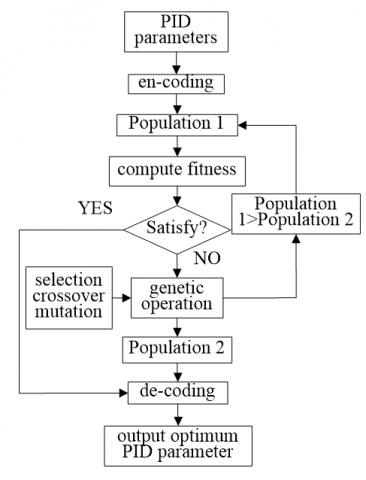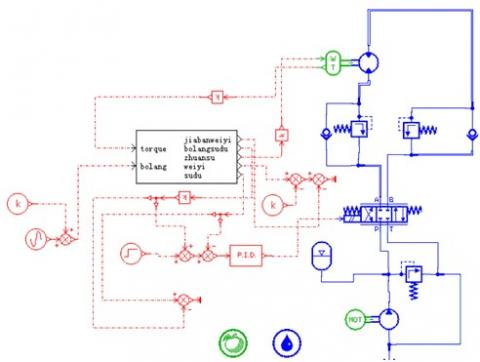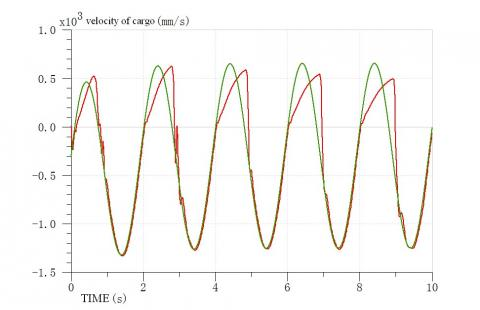# Modeling and Simulation Research of Active Heave Compensation System

Modeling and Simulation Research of Active Heave Compensation System

Mian Dong Yougang SunHaiyang Qiang Xiaotian Wei

College of Logistic Engineering,Shanghai Maritime University, Shanghai, China

Corresponding Author Email:
yoga.sun@qq.com
Page:
15-18
|
DOI:
http://dx.doi.org/10.18280/rces.010204
|
Accepted:
|
Published:
30 December 2014
| Citation

OPEN ACCESS

Abstract:

In order to eliminate the potential safety hazard of the wave in vertical direction when hoisting cargo on the sea, an active heave compensation system (AHCS) is presented for a ship crane. A genetic algorithm based PID controller is put forward to optimize the quality the active heave compensation system (AHCS). In the environment of ADAMS and AMESim, a simulation model of the AHCS is built, which is hydraulic and electro-mechanical integrated system. The simulation results show that the control strategy with the genetic algorithm optimizes the dynamic characteristics, improves the rapidity and the stability of the AHCS and eventually ensures the safety of hoisting cargos on the sea.

Keywords:

Heave compensation, Genetic algorithm, Ship crane, Simulation

1. Introduction

Because of the deep flow, strong storms and the water power, it would generate a 6-degree swaying motion when the ship crane is doing a supply operation for another ship, which would have a relative motion between the ship crane and the being replenishment ship. On one hand, it would cause collision between the lowering cargos and the rising ship. On the other hand, it would also make the cargos on deck hang in the air again. Therefore, we need to design a heave compensation system for the ship crane to ensure the control system is precise enough to meet the need of supplement [2-4].

The genetic algorithm based on the principle of the survival of fittest is a kind of optimization algorithm with embarrassingly parallel, randomness and additivity. The genetic algorithm has two outstanding characteristics which includes the implicit parallelism and the global search of the solution space. Besides the genetic operator, it also includes some key technology such as encoding, the initial population and the fitness function. Compared with the normal PID method, it’s an effective way to adjust the PID parameters by using genetic algorithm based PID method.

In this paper, we find a way, the genetic algorithm based PID, to adjust the PID parameters to control the interference with the cargos form the wave. And we have proved and simulated it in AMESim.

2. The Conventional PID Control

In an automation control system, PID, which is also known as Proportional Integral Differential, is a kind of normal control law in controllers. PID control is to constitute a control volume to control the controlled object through the linear combination of proportion, integral calculus and differential calculus of deviation.

The relation between output u(t) and input e(t) in the PID controller can be expressed as below.

$u(t)=K_{p}\left[e(t)+\frac{1}{T_{i}} \int e(t) d t+T_{d} \frac{d e(t)}{d t}\right]$        (1)

Where $k_{p}$ is the proportion factor, $k_{i}$ is the integral time constant, $k_{d}$ is the derivative time constant.

Because of the uncertain parameters, poor performances and poor adaptabilities, the conventional PID controllers can not achieve ideal control effect and even limit the application of the PID controller.

3. Genetic Algorithms

GA, genetic algorithms, is a method which is based on the natural selection and genetic theoretic and combines the survival of the fittest rules in the process of biological evolution with the random information exchange mechanism in the chromosome [5-6].

GA imitates the biogenic and the steps of the evolution, and also introduces some methods such as reproduction, crossover and mutation. The reproduction is to pick up the sample which possesses a higher fitness from parent population, and then according to the survival of the fittest rules, to inhibit or even eliminate the samples with lower fitness form offspring. Crossover is to pick up a random string from mating pool, and generate a cross at random. Exchange each bit after the cross and generate a new sample, which will greatly increase the space GA would search. Mutation is to simulate the biological genetic mutation. = Because reproduction and crossover can not ensure the reservation of the important genetic information, mutation is being introduced.

4. PID Parameter Optimization Based on GA

We describe the heave compensation control process as a the first-order inertia model with FOPDT. The transfer function is showed as below

$W(S)=\frac{k}{1+T S} e^{-\tau s}$                    (2)

Where k is the amplification coefficient, T is the approximate time constant, $\tau$ is the pure time delay of the transfer function of the controlled process.

The system structure chart with the PID controller is showed in Fig1.

## 1.pngFigure 1. Control structure of PID controller

Formula in the PID controller is showed as below.

$u(t)=K_{p}\left[e(t)+\frac{1}{T_{i}} \int e(t) d t+T_{d} \frac{d e(t)}{d t}\right]$             (3)

To discretize the formula just mentioned before.

$u(k)=K_{p}\left[e(k)+\frac{T_{1}}{T_{i}} \sum_{i=0}^{k} e(i)+T_{d} \frac{e(k)-e(k-1)}{T_{i}}\right.$

$=K_{p}\left[e(k)+K_{i} T_{i} \sum_{i=0}^{k} e(i)+K_{d} \frac{e(k)-e(k-1)}{T_{1}}\right]$      (4)

Where $\mathrm{T}_{1}$ is the sampling period,$K_{i}=K_{P} / T_{i}$, $K_{d}=K_{p} T_{d}$, $K_{p}, T_{i}, T_{d}$ are the PID control parameters, which are equal to $K_{p}, K_{i}, K_{d}$.

In this paper, we take equation 4 as the controlled objects and make study of how to use GA to optimize the PID parameters.

1) Coding and decoding

When using GA, the first problem we will meet is to code and decode the parameters. It’s a multi-parameter optimization problem in this paper, so we have to use binary code. In order to take the search space and the search efficiency into account, we use five unsigned binary codes to express each parameter. Three of them are concatenated together and become a single sample.

We can use the equation showed below to get parameter $k_{p}$.

$K_{p}=\frac{(2-0.01) r}{2^{5}-1}+0.01=\frac{1.99 r}{31}+0.01$                          (5)

Equation 5 is the decoding equation for the parameter $k_{p}$. The other two parameters also can be worked out.

2) Determination of the fitness function

In fact, the fitness function is the other form of the objective function. There are lots of forms of the parameter optimization for PID controller, and we choose the objective function as below.

$J=\int_{0}^{\infty} t|e| d t$            (6)

To discretize the equation above, we will get

$J=\sum_{i=0}^{Q} t_{i}|e(i)|$         (7)

Q is determined by the sample period $T_{1}$ and the setting time.The genetic operation is according to the fitness value. And the fitness value is non-negative. The optimization direction of the objective function should correspond to the direction of the fitness value. So, we turn the objective function $F=\left\{\begin{array}{c}F_{\max }-J, \quad J<F_{\max } \\ 0, \text { others }\end{array}\right.$ into the fitness function

$F=\left\{\begin{array}{c}F_{\max }-J, \quad J<F_{\max } \\ 0, \text { others }\end{array}\right.$

$F=\left\{\begin{array}{c}F_{\max }-J, \quad J<F_{\max } \\ 0, \text { others }\end{array}\right.$          (8)

$F_{\max }$ is a maximum of the objective function in the current population.

3) Terminating conditions of genetic operations

The classical method is to set a maximum iteration. It’s over when it reaches the set maximum iteration.

The process of GA is showed as follows.

## 2.pngFigure 2. Flow chart of GA

5. Modeling and Simulation

AMESim is a modeling and simulation platform for multi-disciplinary field of complex systems, which provides the graphical modeling of hydraulic system, control system,mechanical systems and other fields to free the users from the tedious mathematical modeling and to focus on the design of the system itself.

Importing the defined ADAMS mechanical motion module into the AMESim, the Control Model of Heave Compensation is established (Figure 3). Set parameters for each component, motor speed: 1500 rev/min; storage capacity: 1000L; servo valve rated flow: 1000L; servo valve natural frequency: 80HZ; servo valve damping ratio: 0.8; hydraulic motor speed: 100rev/min; balance valve pressure: 20bar.

## 3.jpgFigure 3. Simulation model

6. The Simulation Results

To illustrate the superiority of the genetic algorithm to optimize the parameters presented in this paper, a conventional PID controller is used to do comparative experiment. Design Exploration Tool of AMESim is used to optimize the three parameters($k_{p}, k_{i}, k_{d}$) of PID controller by genetic algorithm. Set the number of population to 40, regeneration rate to 0.8, the maximum evolution generation to 20, the likelihood of mutation to 0.5, mutation amplitude to 0.2, the number of seed to 1. Conventional PID control system parameters are taken as: Kp= 0.001, Ki = 0.,7, Kd= 0.0002. The input of speed command is -300mm/s.The sine function of  the frequency of 0.5 HZ and  the amplitude of 1000 mm is set for the relative displacement between the receiving ship and the cargo.

Run the simulation. Figure 4 shows the response curve of cargo velocity of the electro-hydraulic servo system with conventional PID controller and Figure 5, with the genetic algorithm PID controller.

## 4.jpgFigure 4. Conventional PID controller

## 5.jpgFigure 5. Genetic algorithm PID controller

By comparison, the delay of following command signal of the electro-hydraulic servo system with conventional PID controller is about 0.48 s, which reveals response speed is too slow and the curve is not smooth. But to the electro-hydraulic servo system with the genetic algorithm PID controller, the delay of following command signal reduces to 0.29 s. And the curve is smoother. As a result, the dynamic performance of heave compensation control system has been significantly improved by using the genetic algorithm PID controller.

7. Conclusion

This paper use genetic algorithm PID self-tuning method to control the vertical loading of the offshore crane. A simulation model of electro-mechanical and hydraulic integrated system has established in AMESim. Simulation results reveal that genetic algorithm PID controller has better effect and stronger adaptability, and the control performance of the heave compensation system is improved. Meanwhile, the genetic algorithm and strategy presented in this paper with general, not only can be used for the optimization of the PID controller parameters of the heave compensation system, but also can be generalized to the PID control optimization of other objects as well as to the optimization problems of a wider range.

Acknowledgment

College Students in Shanghai Innovation Training Project (CXS201405024)

References

1. DONG Da-Shan, SUN You-Gang, LIU Long, Simulation Studies on Dynamic Characters of Floating Cranes, Science Technology and Engineering, vol.13, no.20, pp.5872-5877, 2013.

2. K. Do and J. Pan, Nonlinear Control of an Active Heave Compensation System, Ocean Eng., vol.35, no.5/6, pp.558–571, 2008.

3. J. Neupert, T. Mahl, B. Haessig, O. Sawodny and K. Schneider, A Heave Compensation Approach for Offshore Cranes, Proc. Amer. Control Conf., pp.538–543, 2008.

4. L. Marconi, A. Isidori, and A. Serrani, Autonomous Vertical Landing on an Oscillating Platform: An Internal-Model Based Approach, Automatica, vol. 38, no. 1, pp.21–32, 2002.

5. Larragana P., Poza M., Structure Learning of Bayesian Networks by Genetical Algorithms, Studies in Classification, Data Analysis, and Knowledge Organization, Springer Verlag, pp.300-306, 2006.

6. Yu. I. Minkin and A. I. Petrov, A Self-Organizing Genetical Gorithm, Journal of Computer and Systems Sciences Informational, Vol.40, No.3, pp.416-424, 2006.